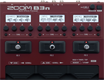# Factory : Comp&Drv

Discussion in 'Zoom B3n' started by Tonelib, May 20, 2018.

1. Factory : Comp&DrvDevice: Zoom B3n
Firmware: 2.00

Name on device: Comp&Drv
Optimized for: Phones/Speaker

Effects chain:Combining DYN Comp and D.I Plus, this drive sound can be used in multis.

Effect: "DYN Comp" (Dynamics), active - "yes"
"Sense" = 5
"Attack" = Slow
"Tone" = 47
"Volume" = 53

Effect: "D.I Plus" (Overdrive / Distortion), active - "yes"
"Bass" = 55
"Middle" = 85
"Treble" = 63
"Color" = On
"Cl Volume" = 53
"Blend" = 63
"Gain" = 34
"Ds Volume" = 61

Effect: "BassGEQ" (Filter), active - "yes"
"50Hz" = 0.0
"120Hz" = 0.0
"400Hz" = 0.0
"500Hz" = 4.0
"800Hz" = 2.0
"4.5kHz" = 0.0
"10kHz" = 0.0
"Volume" = 80
"50Hz" = 0.0
"120Hz" = 2.0
"400Hz" = 0.0
"500Hz" = 2.0
"800Hz" = 3.5
"4.5kHz" = -3.0
"10kHz" = 0.0
"Volume" = 77
"Parameter set" = B

Effect: "ZNR" (Dynamics), active - "yes"
"Detect" = GTRIN
"Depth" = 100
"Threshold" = 30
"Decay" = 0

Patch Volume: 100